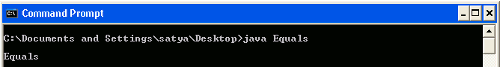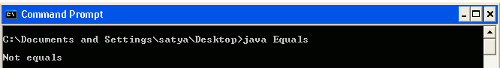# Difference between equals() and == ?

This tutorials will help you to find the difference between equals() and "==" operator.

This tutorials will help you to find the difference between equals() and "==" operator.

## Difference between equals() and == ?

In this section you will find the difference between equals() and ==. When we create a object using new keyword then it has both location in the memory and object state depending on value. Both equals() and "= =" are used to compare object to check equality. But one more difference is that equals() is a method and "= =" is an operator.

The "= =" operator

In java equality operator is used to compare two object. It check to see that both object are from same place in the memory or not. In other word it will check that both object are having same references to the memory location. The example will clarify this:

```public class Equals {
public static void main(String args[])
{
Object ob1=new Object();
Object ob2=new Object();
if(ob1==ob2)
System.out.println("Equals");
else
{
System.out.println("Not equals");
}
}
}```So, you might think that it will print "equals" but if you did then you are wrong actually because it will print "Not equals". But the same code if you write as below program then it will print "equals" to the console.

```public class Equals {
public static void main(String args[])
{
Object ob1=new Object();
Object ob2=ob1;
if(ob1==ob2)
System.out.println("Equals");
else
{
System.out.println("Not equals");
}
}
}```

In the above code, obj1 and obj2 both  references to the same memory location because of the statement Object ob2=ob1 and then output is as follows:The equals() method :

We have discussed the equality operator, Now lets discuss about equals() method in java. The equals() method which is defined in object class. equals() method behave same as the equality operator but rather then checking the memory location, equals() method will compare the content of two object whether it is same or not. The java String class overrides the equals() implementation in the object class and its overrides the method to check the content of the string object character by character whether it is equal or not.

```public class Equals {
public static void main(String args[])
{
Object ob1=new Object();
Object ob2=new Object();
if(ob1.equals(ob2))
System.out.println("Equals");
else
{
System.out.println("Not equals");
}
}
}```

As we discussed equals() method compare the content of the string object, so it will compare ob1 and ob2 content are same or not. The output of the program is:Ads